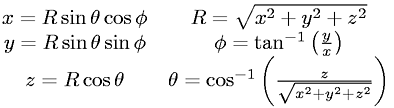Equations > Geometry > Coordinate Systems > Cartesian to Spherical Coordinates (3-D)

Cartesian to Spherical Coordinates (3-D)Latex Code:

MathML Code:

 $\begin{array}{ccc}\hfill x=R\mathrm{sin}\theta \mathrm{cos}\phi \hfill & \hfill R=\sqrt{{x}^{2}+{y}^{2}+{z}^{2}}\hfill & \hfill \hfill \\ \hfill y=R\mathrm{sin}\theta \mathrm{sin}\phi \hfill & \hfill \phi ={\mathrm{tan}}^{-1}\left(\fracyx\right)\hfill & \hfill \hfill \\ \hfill z=R\mathrm{cos}\theta \hfill & \hfill \theta ={\mathrm{cos}}^{-1}\left(\fracz\sqrt{{x}^{2}+{y}^{2}+{z}^{2}}\right)\hfill & \hfill \hfill \end{array}$

MathType 5.0: